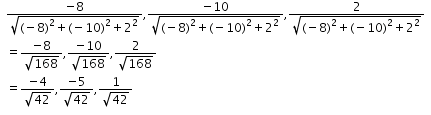# RD Sharma Solutions Class 12 Direction Cosines And Direction Ratios Exercise 27.1

RD Sharma Solutions for Class 12 Maths Chapter 27 Direction Cosines and Direction Ratios Exercise 27.1, is provided here. Tutors at BYJU’S have designed the solutions in a unique and simple method where any student can understand the topics clearly. Here, the RD Sharma Class 12 Solutions to this exercise are provided in pdf format, which can be downloaded easily from the links given below.

## Download PDF of Rd Sharma Solution for Class 12 Maths Chapter 27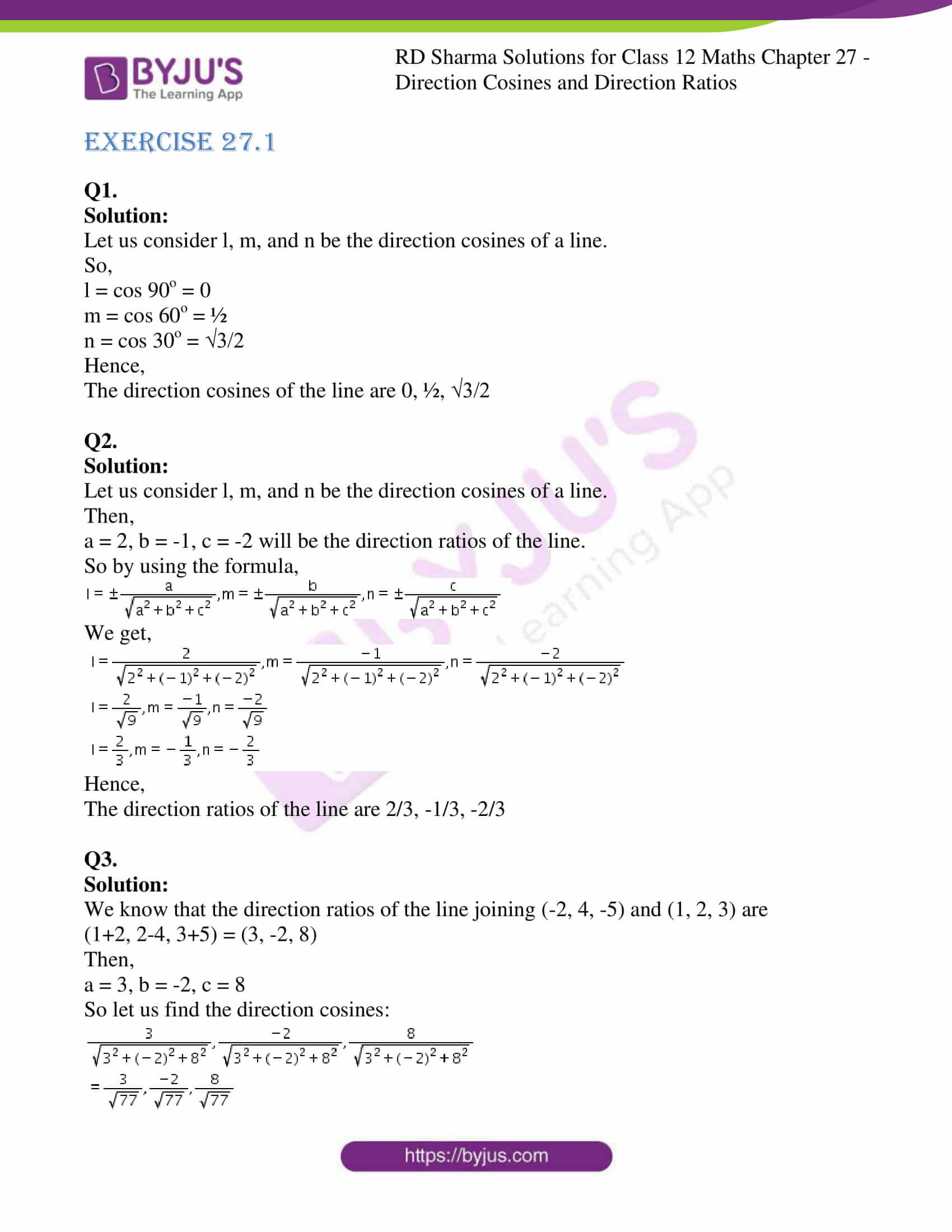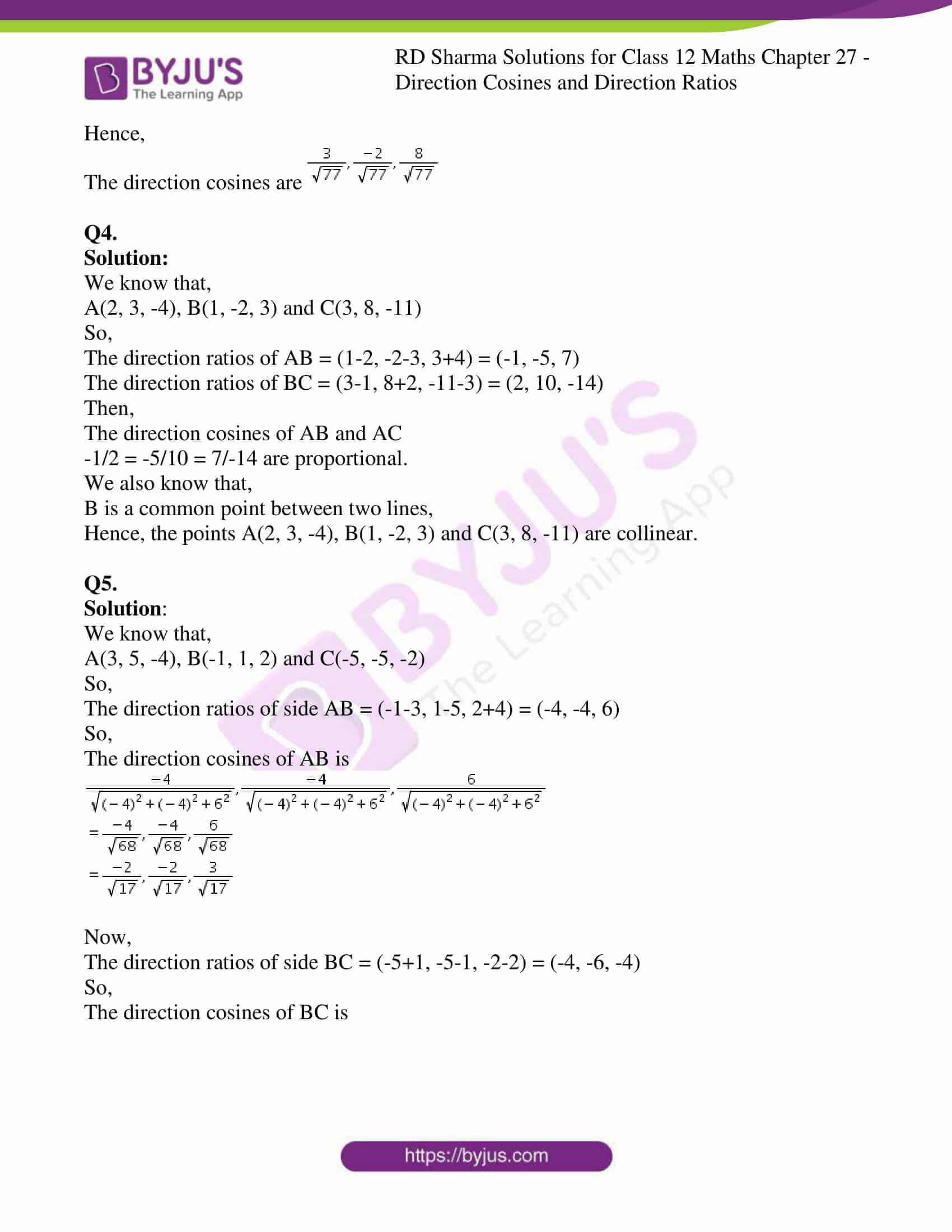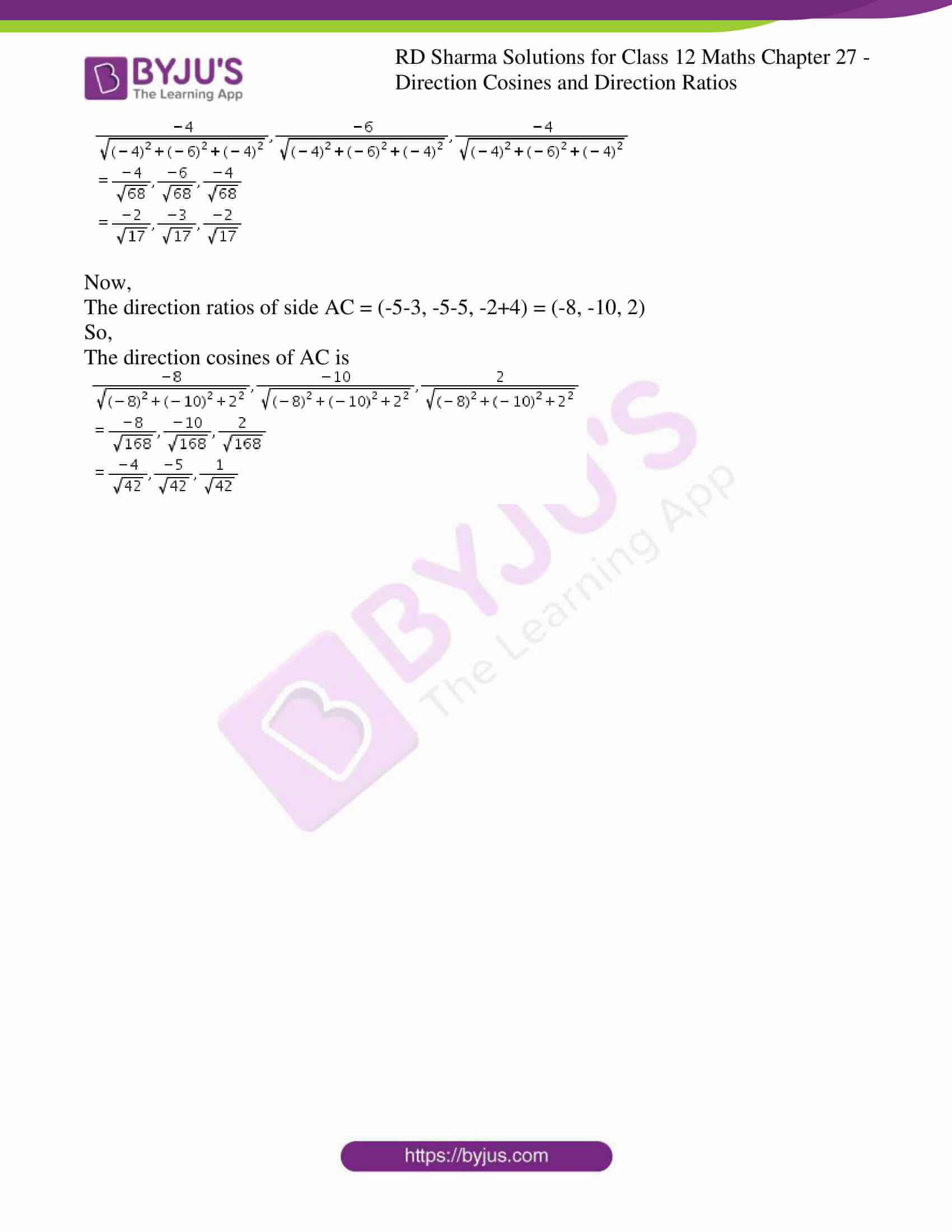### Access Answers for Rd Sharma Solution Class 12 Maths Chapter 27

EXERCISE 27.1

Q1.

Solution:

Let us consider l, m, and n be the direction cosines of a line.

So,

l = cos 90o = 0

m = cos 60o = ½

n = cos 30o = √3/2

Hence,

The direction cosines of the line are 0, ½, √3/2

Q2.

Solution:

Let us consider l, m, and n be the direction cosines of a line.

Then,

a = 2, b = -1, c = -2 will be the direction ratios of the line.

So by using the formula,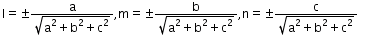We get,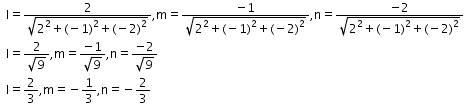Hence,

The direction ratios of the line are 2/3, -1/3, -2/3

Q3.

Solution:

We know that the direction ratios of the line joining (-2, 4, -5) and (1, 2, 3) are

(1+2, 2-4, 3+5) = (3, -2, 8)

Then,

a = 3, b = -2, c = 8

So let us find the direction cosines: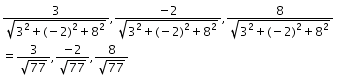Hence,

The direction cosines are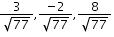Q4.

Solution:

We know that,

A(2, 3, -4), B(1, -2, 3) and C(3, 8, -11)

So,

The direction ratios of AB = (1-2, -2-3, 3+4) = (-1, -5, 7)

The direction ratios of BC = (3-1, 8+2, -11-3) = (2, 10, -14)

Then,

The direction cosines of AB and AC

-1/2 = -5/10 = 7/-14 are proportional.

We also know that,

B is a common point between two lines,

Hence, the points A(2, 3, -4), B(1, -2, 3) and C(3, 8, -11) are collinear.

Q5.

Solution:

We know that,

A(3, 5, -4), B(-1, 1, 2) and C(-5, -5, -2)

So,

The direction ratios of side AB = (-1-3, 1-5, 2+4) = (-4, -4, 6)

So,

The direction cosines of AB is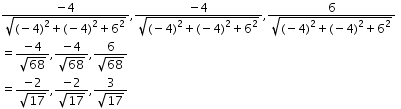Now,

The direction ratios of side BC = (-5+1, -5-1, -2-2) = (-4, -6, -4)

So,

The direction cosines of BC is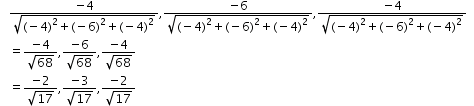Now,

The direction ratios of side AC = (-5-3, -5-5, -2+4) = (-8, -10, 2)

So,

The direction cosines of AC is